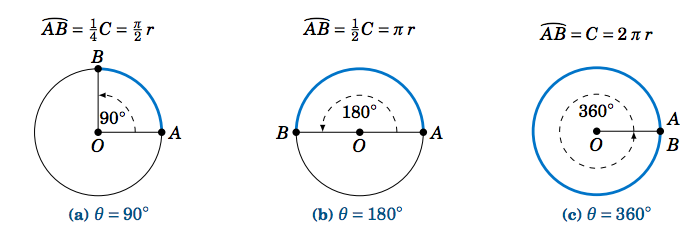$$\newcommand{\id}{\mathrm{id}}$$ $$\newcommand{\Span}{\mathrm{span}}$$ $$\newcommand{\kernel}{\mathrm{null}\,}$$ $$\newcommand{\range}{\mathrm{range}\,}$$ $$\newcommand{\RealPart}{\mathrm{Re}}$$ $$\newcommand{\ImaginaryPart}{\mathrm{Im}}$$ $$\newcommand{\Argument}{\mathrm{Arg}}$$ $$\newcommand{\norm}{\| #1 \|}$$ $$\newcommand{\inner}{\langle #1, #2 \rangle}$$ $$\newcommand{\Span}{\mathrm{span}}$$

4.1: Radians and Degrees

•• Contributed by Michael Corral
• Professor (Mathematics) at Schoolcraft College

$$\newcommand{\vecs}{\overset { \rightharpoonup} {\mathbf{#1}} }$$

$$\newcommand{\vecd}{\overset{-\!-\!\rightharpoonup}{\vphantom{a}\smash {#1}}}$$Figure 4.1.1 Angle $$θ$$ and intercepted arc $$\overparen{AB}$$ on circle of circumference $$C = 2πr$$

In Figure 4.1.1 we see that a central angle of $$90^\circ$$ cuts off an arc of length $$\tfrac{\pi}{2}\,r$$, a central angle of $$180^\circ$$ cuts off an arc of length $$\pi\,r$$, and a central angle of $$360^\circ$$ cuts off an arc of length $$2\pi\,r$$, which is the same as the circumference of the circle. So associating the central angle with its intercepted arc, we could say, for example, that
$\nonumber 360^\circ \quad\text{"equals''}\quad 2\pi\,r \quad\text{(or $$2\pi$$ 'radiuses').}$
The radius $$r$$ was arbitrary, but the $$2\pi$$ in front of it stays the same. So instead of using the awkward "radiuses'' or "radii'', we use the term radians:
$\label{4.1} \boxed{360^\circ ~=~ 2\pi ~~\text{radians}}$
The above relation gives us any easy way to convert between degrees and radians:

\begin{alignat}{3} \textbf{Degrees to radians:}&\quad x~~\text{degrees}\quad&=\quad \left( \frac{\pi}{180} \;\cdot\; x \right) ~~\text{radians}\label{eqn:deg2rad}\\ \textbf{Radians to degrees:}&\quad x~~\text{radians}\quad&=\quad \left( \frac{180}{\pi} \;\cdot\; x \right) ~~\text{degrees}\label{eqn:rad2deg} \end{alignat}

Equation \ref{eqn:deg2rad} follows by dividing both sides of Equation \ref{4.1} by $$360$$, so that $$1^\circ = \frac{2\pi}{360} = \frac{\pi}{180}$$ radians, then multiplying both sides by $$x$$. Equation \ref{eqn:rad2deg} is similarly derived by dividing both sides of Equation \ref{4.1} by $$2\pi$$ then multiplying both sides by $$x$$.

The statement $$\theta = 2\pi$$ radians is usually abbreviated as $$\theta = 2\pi$$ rad, or just $$\theta = 2\pi$$ when it is clear that we are using radians. When an angle is given as some multiple of $$\pi$$, you can assume that the units being used are radians.

Example 4.1

Convert $$18^\circ$$ to radians.

Solution:

Using the conversion Equation \ref{eqn:deg2rad} for degrees to radians, we get

$\nonumber 18^\circ ~=~ \frac{\pi}{180} \;\cdot\; 18 ~=~ \boxed{\frac{\pi}{10} ~~\text{rad}} ~.$

Example 4.2

Convert $$\frac{\pi}{9}$$ radians to degrees.

Solution:

Using the conversion Equation \ref{eqn:rad2deg} for radians to degrees, we get

$\frac{\pi}{9} ~~\text{rad} ~=~ \frac{180}{\pi} \;\cdot\; \frac{\pi}{9} ~=~ \boxed{20^\circ} ~.\nonumber$

Table 4.1 Commonly used angles in radiansTable 4.1 shows the conversion between degrees and radians for some common angles. Using the conversion Equation \ref{eqn:rad2deg} for radians to degrees, we see that

$\nonumber 1 ~~\text{radian} ~~=~~ \frac{180}{\pi}~~\text{degrees} ~~\approx~~ 57.3^\circ ~.$

Formally, a radian is defined as the central angle in a circle of radius $$r$$ which intercepts an arc of length $$r$$, as in Figure 4.1.2. This definition does not depend on the choice of $$r$$ (imagine resizing Figure 4.1.2).

One reason why radians are used is that the scale is smaller than for degrees. One revolution in radians is $$2\pi \approx 6.283185307$$, which is much smaller than $$360$$, the number of degrees in one revolution. The smaller scale makes the graphs of trigonometric functions (which we will discuss in Chapter 5) have similar scales for the horizontal and vertical axes. Another reason is that often in physical applications the variables being used are in terms of arc length, which makes radians a natural choice.

The default mode in most scientific calculators is to use degrees for entering angles. On many calculators there is a button labeled $$\fbox{\( DRG$$}\) for switching between degree mode (D), radian mode (R), and gradian mode (G). On some graphing calculators, such as the the TI-83, there is a $$\fbox{\(MODE$$}\) button for changing between degrees and radians. Make sure that your calculator is in the correct angle mode before entering angles, or your answers will likely be way off. For example,

\nonumber \begin{align*} \sin\;4^\circ ~&=~ \phantom{-}0.0698 ~,\\ \nonumber \sin\;(4~\text{rad}) ~&=~ -0.7568 ~, \end{align*}

so the values are not only off in magnitude, but do not even have the same sign. Using your calculator's $$\fbox{\(\sin^{-1}$$}\), $$\fbox{\(\cos^{-1}$$}\), and $$\fbox{\(\tan^{-1}$$}\) buttons in radian mode will of course give you the angle as a decimal, not an expression in terms of $$\pi$$.

You should also be aware that the math functions in many computer programming languages use radians, so you would have to write your own angle conversions.